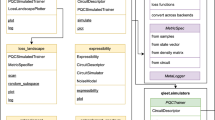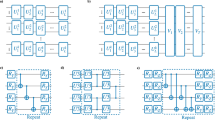# Estimating the degree of non-Markovianity using variational quantum circuits

## Abstract

Several applications of quantum machine learning (QML) rely on a quantum measurement followed by training algorithms using the measurement outcomes. However, recently developed QML models, such as variational quantum circuits (VQCs), can be implemented directly on the state of the quantum system (quantum data). Here, we propose to use a qubit as a probe to estimate the degree of non-Markovianity of the environment. Using VQCs, we find an optimal sequence of qubit-environment interactions that yield accurate estimations of the degree of non-Markovianity for the amplitude damping, phase damping, and the combination of both models. This work contributes to practical quantum applications of VQCs and delivers a feasible experimental procedure to estimate the degree of non-Markovianity.

This is a preview of subscription content, log in via an institution to check access.

Price excludes VAT (USA)
Tax calculation will be finalised during checkout.

### Similar content being viewed by others### qLEET: visualizing loss landscapes, expressibility, entangling power and training trajectories for parameterized quantum circuits### Optimal quantum state transformations based on machine learning

Tian-Hui Zhao, Ming-Hao Wang & Bin Zhou### Classical splitting of parametrized quantum circuits

Cenk Tüysüz, Giuseppe Clemente, … Karl Jansen

## Availability of data and materials

The data that support the findings of this study are available from the corresponding author upon reasonable request.

1. For the PD channel, using $$\check{\mathcal {N}}=\text {max}\left( w_0+w_1\langle \sigma _z\rangle ,0\right)$$ as the estimate of the degree of non-Markovianity, we obtained $$1.7\times 10^{-6}$$ for the MSE over the test data.

## Acknowledgements

The authors thank Mauro Cirio for helpful comments on the manuscript. H.T.D. acknowledges support from Universidad Mayor through a postdoctoral fellowship. D.T acknowledges financial support from Universidad Mayor through the Doctoral fellowship. F.F.F. acknowledges support from Fundacão de Amparo á Pesquisa do Estado de São Paulo (FAPESP), Project No. 2019/05445-7. A. N. acknowledges financial support from Fondecyt Iniciación No 11220266. R.C. acknowledges financial support from FONDECYT Iniciación No. 11180143.

## Author information

Authors

### Contributions

H.T.D., R.C., and D.T. conceptualized the idea. F.F.F. provided the dataset for PD and AD channels. A.N. advised on the experimental implementations of the proposed scheme. H.T.D. performed the numerical simulations. All authors discussed the results and contributed to writing and review of the paper

### Corresponding author

Correspondence to Hossein T. Dinani.

## Ethics declarations

### Conflict of interest

The authors declare no competing interests.

### Publisher's Note

Springer Nature remains neutral with regard to jurisdictional claims in published maps and institutional affiliations.

## Appendices

Here, following Ref. (Ruder 2016), we give some details about the Adagrad optimizer that we used in our simulations. Adaptive gradient (Adagrad) is a variation of the gradient descent (GD) optimizer. In the GD algorithm, the parameters, labeled by $$\varphi$$ here, are updated in the opposite direction of the gradient of the cost function $$C(\varphi )$$. In other words, for every parameter $$\varphi _i$$ at each time step t, the update rule can be written as

\begin{aligned} \varphi _{t+1,i}=\varphi _{t,i}-\eta \nabla _{\varphi _i}C(\varphi ), \end{aligned}
(A1)

where $$\eta$$ is the learning rate, which is assumed to be constant and independent of $$\varphi _i$$ thorough the learning process.

In Adagrad, a different learning rate is used for every parameter $$\varphi _i$$ at every time step. In the update rule for Adagrad, the learning rate at each time step t for every parameter $$\varphi _i$$ is based on the past gradients that have been calculated for $$\varphi _i$$ (Ruder 2016)

\begin{aligned} \varphi _{t+1,i}=\varphi _{t,i}-\frac{\eta }{\sqrt{g_{t+1,i}+\varepsilon }}\nabla _{\varphi _i}C(\varphi ). \end{aligned}
(A2)

Here, $$g_{t+1,i}$$ is the sum of the squares of the gradients with respect to $$\varphi _i$$ up to time step $$t+1$$,

\begin{aligned} g_{t+1,i}=g_{t,i}+\left( \nabla _{\varphi _i}C(\varphi )\right) ^2, \end{aligned}
(A3)

where $$g_0=0$$, and $$\varepsilon$$ (usually chosen on the order of $$10^{-8}$$) is for avoiding division by zero.

The weakness of Adagrad is the accumulation of the squared gradients in the denominator. The accumulated sum keeps growing during the training process as the added terms are all positive. As a result, the learning rate could approach zero, and therefore, the algorithm stops learning. To avoid this issue in our simulations, when the rate of learning became very small, we reinitialized the optimization process with the newly found hyperparameters as the initial values.

### Appendix B. RMSprop

Root mean square propagation (RMSprop) is a variation of Adagrad algorithm which uses a decaying average of squared gradients in the adaptation of the step size for each parameter (Ruder 2016). The use of a decaying average allows the algorithm to forget early gradients and only focus on the most recent gradients during the optimization process. As a result, RMSprop overcomes the AdaGrad’s diminishing learning rates. In RMSprop, the parameter update rule is

\begin{aligned} \varphi _{t+1,i}=\varphi _{t,i}-\frac{\eta }{\sqrt{g_{t+1,i}+\varepsilon }}\nabla _{\varphi _i}C(\varphi ). \end{aligned}
(B1)

In this case, we have

\begin{aligned} g_{t+1,i}=\gamma g_{t,i}+(1-\gamma )\left( \nabla _{\varphi _i}C(\varphi )\right) ^2, \end{aligned}
(B2)

where $$g_{0}=0$$, and for $$\gamma$$ it is suggested to use $$\gamma =0.9$$ (Hinton et al. 2012).

In our simulations, using RMSprop algorithm, a local minima of the cost function reached quickly (in less number of steps than Adagrad). However, the algorithm then started to diverge, resulting in large values for the cost function. Therefore, once RMSprop reached the local minima, we followed the optimization process with Adagrad to reach the desired accuracy.

## Rights and permissions

Springer Nature or its licensor (e.g. a society or other partner) holds exclusive rights to this article under a publishing agreement with the author(s) or other rightsholder(s); author self-archiving of the accepted manuscript version of this article is solely governed by the terms of such publishing agreement and applicable law.

Reprints and Permissions